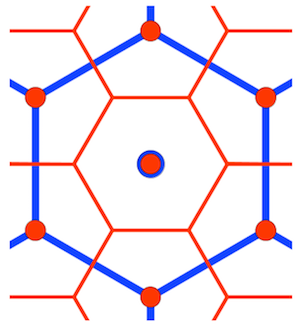This operation is performed by function h3ToGeoBoundary. See the comments in the function source code for more detail.• The function _faceIjkToGeoBoundary calculates the ijk coordinates of the cell center point in the appropriate substrate grid (determined in the last step), and each of the substrate vertices is translated using the cell center point ijk. Each vertex ijk is then transformed onto the appropriate face and Hex2d coordinate system using the approach taken in finding a cell center point. If adjacent vertices lie on different icosahedron faces a point is introduced at the intersection of the cell edge and icosahedron face edge.
• The Hex2d coordinates are then converted to latitude/longitude using _hex2dToGeo.